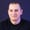FREELessons: 20Length: 1.2 hours• Overview
• Transcript

# 5.1 CSS Triangles

Making shapes in CSS is really easy. We all know to make a square or a rectangle right? But what about triangles?

Well, let’s find out.

## 7.Conclusion1 lesson, 01:47

### 5.1 CSS Triangles

Making shapes in CSS is really easy. We all know how to make a square or a rectangle right? But what about triangles? Well, let's find out. Let me show you the setup first. We have four divs with a class of arrow. It's a common class, and then additional classes for up, down, left, and right. So, in order to create triangles, first you gotta set the height and the width of that element to zero. So let's target the arrow class, gonna do height 0. I'm gonna do width 0, and also let's do a margin-bottom of 1em, just to separate them a little bit. All right, next up, let's do with the arrow up. Now, the trick here is to use borders. And on an arrow pointing up, for example, you gotta set the left and right borders to transparent while you set the opposing border to a solid color. So let me show you. We'll do border-left 10 pixels solid transparent. Same goes for border-right and then border-bottom. Again, 10 pixels, solid, let's make this one red. Save. And that is a nice triangle. If you want to create a triangle pointing down, well it's pretty much the same thing. Let's copy the up, down, border-left and right will be exactly the same. But the opposite border, which is border-up, border-top sorry, will be 10 pixels solid. Let's make this blue. And now it's pointing in the other direction. For left and right, it's very similar, except we won't make the left and right borders transparent. Instead, we'll make the top and bottom. So, that's gonna look something like this. Let's start with left, so we're gonna say border-top. 10 pixels solid transparent, border-bottom exactly the same. And then border-right, 10 pixels solid, let's make it green. And that's an arrow pointing to the left. And to the right it's exactly the same principle, right here and left here. And let's make this grey. Okay, perfect. Now if you take a closer look, you'll see that these triangles don't have equal sides. Now to make that happen, we've got to use a special formula which looks something like this. Let's take the up border, the up triangle, sorry. So on the border-bottom, we've got to set the border size to the following formula. So let's do this. Border-left-width + border-right-width, all of this, multiplied by 0.866. So if we do some quick math here, well, we have 10 plus 10, that's 20, multiplied by 0.866, and that will give us 17.32. So we'll just put 17 pixels here. Okay, and right now we have a triangle with equal sides. Now once you make the triangles, you can also rotate them like this using some simple transform. So let's grab this last triangle right here. Let's make it a bit bigger, like 30 pixels. And we're gonna do this webkit-transform: rotate, let's do by 90 degrees. Okay, so this is how it looks like. Save, and now it's pointing down. And of course, we can add all the other vendor prefixes right here like mso, mos, and of course the default, transform method rotate 90 degrees. All right, so there you have it, a very simple method of creating triangles in CSS.

Back to the top